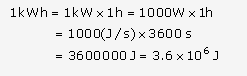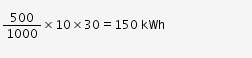# Define commercial unit of energy. Derive relationship between this unit

Define commercial unit of energy. Derive relationship between this unit of energy and SI unit of energy. An electrical device of 500 W is used daily in a household for 10 hours. Calculate the energy consumed in the month of April.

Commercial unit of energy in 1 kWh.
One kilowatt-hour is the amount of energy consumed by an agent in one hour working at a constant rate of one kilowatt (i.e. at the rate of 1000 J in one second).
SI unit of energy is joule.The energy consumed by device of power 500W for 30 days
E = P x t